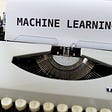# Getting started with Machine Learning Part-2(#GO-ML)

In the previous blog, we discussed Python basics, Pandas basics, numpy basics. If you haven't been through the tutorials please have a look at it.

So let's get started with our second course Getting started with Machine Learning with Python. In this course, we will be discussing the following:-

1. Types of learning in ML
2. Classification(Knn algorithm)

Machine learning is sub-divided into the following types of learning:-

1. Supervised learning
2. Unsupervised Learning
3. Semi-supervised Learning
4. Reinforcement Learning

Now let's have a quick introduction of the above types of learning:-

# Supervised Learning

Supervised learning as the name indicates the presence of a supervisor as a teacher. Basically supervised learning is learning in which we teach or train the machine using data which is well labelled that means some data is already tagged with the correct answer. After that, the machine is provided with a new set of examples(data) so that supervised learning algorithm analyses the training data(set of training examples) and produces a correct outcome from labelled data.

# Unsupervised Learning

Unsupervised Learning is a class of Machine Learning techniques to find the patterns in data. The data given to the unsupervised algorithm are not labelled, which means only the input variables(X) are given with no corresponding output variables.eg.:-clustering, LDA

# Semi-Supervised learning

As you may have guessed, semi-supervised learning algorithms are trained on a combination of labelled and unlabeled data. This is useful for a few reasons. First, the process of labelling massive amounts of data for supervised learning is often prohibitively time-consuming and expensive.

What’s more, too much labelling can impose human biases on the model. That means including lots of unlabeled data during the training process actually tends to improve the accuracy of the final model while reducing the time and cost spent building it.

# Reinforcement Learning

A reinforcement learning algorithm, or agent, learns by interacting with its environment. The agent receives rewards by performing correctly and penalties for performing incorrectly. The agent learns without intervention from a human by maximizing its reward and minimizing its penalty.

Now we shall look upon some classification algorithms of Supervised learning.

We will be focusing on the KNN algorithm that is widely used in classification problems.

# K-Nearest Neighbour

Let's make this algorithm simpler by breaking it down into pieces.

KNN algorithm is very simple its just the Euclidean Distance formula.

Simple Right !!

So let's see how can this algorithm be used for classification. We will be considering the iris dataset for this. Let's have a look at how the iris dataset looks like:

# Our Aim

To classify the species of the iris flower(namely:- setosa, versicolor,virginica).

First, let's get the Euclidean distance into code.

Initially, we set the default of distance equal to 0. Then we are running the loop from 0 to the length-1 of the total size of the dataset. In our case, we have test dataset of length 4. So the length becomes equal to 4. And the loops execute 4 times.

This is how the KNN algorithm works

As we see in the above image there are two classes namely A and B. Suppose we introduce test data. And our ultimate goal is to predict its final label i.e A or B. So we calculate the Euclidean distance of the test data with the other dataset. Suppose this is how our test and train dataset looks like

Now we calculate Euclidean distance between the test and train dataset. This is how the process goes.

And as we see that the Euclidean distance for virginica is less. So our nearest neighbour is virginica. This is how KNN algorithm works.

The above was only for two sets of data think of a bigger dataset like iris dataset that has about 732 rows. So we calculate the distance from each row and then we sort the value in ascending order. Code for sorting in Python

The code, as well as the working example, is available in the below notebook. As well as you can find the whole tutorial in my Github.

Show your love my clapping if you like it. Follow my Machine learning corses in Github.

--

--

--

## More from Abhishek Patnaik

I build product with passion. Follow me for product related blogs.

Love podcasts or audiobooks? Learn on the go with our new app.

## Recommended from Medium## An Intro to PyTorch, Vision Transformer Applications, Scaling Analytics, and Jobs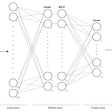## R code and reproducible model development with DVC## Spotting elephants from space: A satellite revolution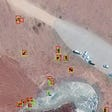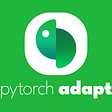## Paper Review and Model Architecture for CNN Classification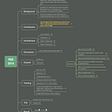## Getting data annotation format right for object detection tasks## Know the Unknown- Discover more from the Data at hand with Machine Learning## Abhishek Patnaik

I build product with passion. Follow me for product related blogs.

## How to implement client and server in Flask for your AI/ML models with code## Histograms in the Context of Data Mining## Example For an Average of Customer Earnings With Rule-Based Classification## Dockerize & Deploy your Machine Learning model